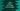# Java program to check if two strings are an anagram or not## Java program to check if two strings are an anagram or not:

A string is called an anagram of another string if the lengths of the strings are equal and one string can be created by rearranging the characters of the another string. In this post, we will write a Java program that will take two strings as inputs from the user and find out if these are anagram or not.

For example, race and palm are anagram strings. Because both strings have the same set of characters and both are of equal length.

### Algorithm to follow:

We will follow the below algorithm in this example:

1. Take the strings as inputs from the user.
2. Check the length of the strings. If the lengths are not equal, return False i.e. these are not anagram strings.
3. If the lengths are equal, convert the strings to lowercase and then convert these to two character arrays.
4. Sort the characters of both arrays.
5. Compare the arrays. If both are equal, then the strings are anagram. Else, these are not anagram.

### Java program:

Let’s write down the complete program:

``````import java.util.Arrays;
import java.util.Scanner;

class Main {

private static boolean isAnagram(String str1, String str2) {
if (str1.length() != str2.length())
return false;

char[] str1Arr = str1.toLowerCase().toCharArray();
char[] str2Arr = str2.toLowerCase().toCharArray();

Arrays.sort(str1Arr);
Arrays.sort(str2Arr);

return Arrays.equals(str1Arr, str2Arr);
}

public static void main(String[] args) {
String str1, str2;
Scanner sc = new Scanner(System.in);

System.out.println("Enter the first string: ");
str1 = sc.nextLine();

System.out.println("Enter the second string: ");
str2 = sc.nextLine();

if (isAnagram(str1, str2)) {
System.out.println("These are anagram.");
} else {
System.out.println("These are not anagram.");
}
}
}``````

Here,

• isAnagram method is used to check if two strings are anagram or not. This method takes two strings as the parameters and returns a boolean value.

• If the length of the strings are not equal, it returns false.
• It converts the strings to lower case and converts them to character arrays.
• By using Arrays.sort method, it sorts the contents of the arrays.
• Arrays.equals is used to check if both character arrays are equal or not. If both are equal, it returns true, else it returns false.

If you run the above program, it will give output as like below:

``````Enter the first string:
save
Enter the second string:
vase
These are anagram.

Enter the first string:
save
Enter the second string:
saave
These are not anagram.

Enter the first string:
save
Enter the second string:
news
These are not anagram.``````